# Bihar Board Class 12th Physics Solutions Chapter 8 Electromagnetic Waves

Bihar Board Class 12 Physics Solutions Chapter 8 Electromagnetic Waves Textbook Questions and Answers, Additional Important Questions, Notes.

## BSEB Bihar Board Class 12 Physics Solutions Chapter 8 Electromagnetic Waves

### Bihar Board Class 12 Physics Electromagnetic Waves Textbook Questions and Answers

Question 1.
Figure shows a capacitor made of two circular plates each of radius 12 cm, and separated by 5.0 cm. The capacitor is being charged by an external source (not shown in the figure). The charging current is constant and equal to 0.15A.
(a) Calculate the capacitance and the rate of change of potential difference between the plates.
(b) Obtain the displacement current across the plates.
(c) Is Kirchhoff’s first rule (junction rule) valid at each plate of the capacitor? Explain.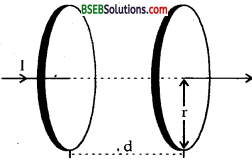Herd, r = radius of circular plates of the capacitor = 12 cm = 12 x 10-2 m.
d spoling between the plates = 5 cm = 5 x 102m.
I = charging current = 0.15 A.
A = Area of plates = πr2
e0 = 8.854 x 10-12 N-1 C2 nr2
.;. A = Area of plates = πr2
= π x (12 x 102)2 m2

(a) C = Capacitance of capacitor = ?
dV = rate of change of potential difference between the plates = ?
Using the relation, C = $$\frac{\varepsilon_{0} \mathrm{~A}}{\mathrm{~d}}$$, we get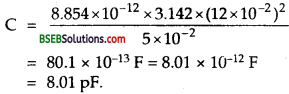We know that the charge on the plates of the capacitor is given by
q = CV
∴ $$\frac{\mathrm{dq}}{\mathrm{dt}}=\mathrm{C} \frac{\mathrm{d} \mathrm{V}}{\mathrm{d} \mathrm{t}}$$ (∵ $$\frac{dq}{dt}$$ = I)
or I = C $$\frac{dV}{dt}$$ , or $$\frac{dV}{dt}$$ = $$\frac{I}{C}$$
∴ $$\frac{dV}{dt}$$ = $$\frac{0.15}{8.01 \times 10^{-12}}$$ = 1.87 x 1010Vs-1(b) Id = displacement current across the plates = ?
We know that
Id = $$\frac{dV}{dt}$$ (ε0 øE) = ε0$$\frac{\mathrm{d} \phi_{\mathrm{E}}}{\mathrm{d} \mathrm{t}}$$
Where øE = electric flux across the loop = $$\oint_{\text {loop }} \overrightarrow{\mathrm{E}} \cdot \overrightarrow{\mathrm{d} S}$$
= EA = $$\frac{\mathrm{q}}{\varepsilon_{0}}$$
Id = ε0$$\frac{d}{dt}$$($$\frac{\mathrm{q}}{\varepsilon_{0}}$$) = $$\frac{\varepsilon_{0}}{\varepsilon_{0}} \cdot \frac{\mathrm{dq}}{\mathrm{dt}}$$ = I
= 0.15 A.
i.e. displacement current is equal to conduction current,
(c) Yes, Kirchhoff’s first rule is valid at each plate of the capacitor if the current is equal to the sum of the conduction current and the displacement current.

Question 2.
A parallel plate capacitor (Fig. below) made of circular ‘ plates each of radius R = 6.0 cm has a capacitance C = 100 pF. The capacitor is connected to a 230 V a.c. supply with a (angular) frequency ; of 300 rad s-1.
(a) What is the rms value of the conduction current?
(b) Is the conduction current equal to the displacement current?
(c) Determine the amplitude of B at a point 3.0 cm from the axis between the plates.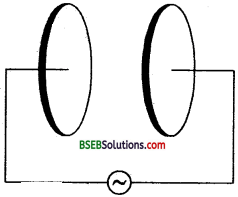Here R = radius of each circular plate of the capacitor = 6 cm = 6 x 102m.
C = 100 pF = 100 x 10-12 F = 10-10 F.
Er.m.s= r.m.s. value of A.C. supply = 230 V ,
ω = angular frequency of A.C. supply = 300 rad s-1.
(a) Ir.m.s = r.m.s. value of conduction current = ? .
We know that= 230 x 300 x 10-10 = 69 x 10-7 A = 6.9 x 10-6 A = 6.9 μA.

(b) Yes, I – I0 always for a parallel plate capacitor whether I is steady d.c. or a.c. (oscillating in time) can be proved as follows :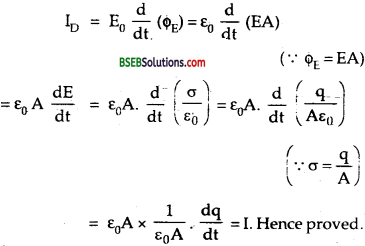(c) We know that the magnetic’ field at a point between the plates is given by according to Ampere’s Circuital law,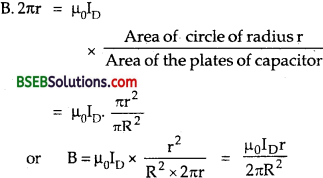This formula is valid even if ID is oscillating. Also we know’ that ID
= I.
∴ B = $$\frac{\mu_{0} \mathrm{Ir}}{2 \pi \mathrm{R}^{2}}$$
If I = I0 = peak value of current, then the amplitude of B = maximum value of B.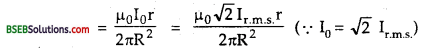Here, Ir.m.s m s = 6.9 x 106 A, r = distance of point from the axis between the plates = 3.0 cm. .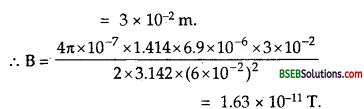Question 3.
What physical quantity is the same for X-rays of wavelength 10-10m, red light of wavelength 6800 A and radiowaves of wavelength 500 m?
X-rays, red light and radio waves all are the electromagnetic waves. They have different wave lengths and frequencies. But the physical quantity which is same for all of these is the velocity of light in vacuum which is denoted by C and is equal to 3 x 108 ms-1.Question 4.
A plane electromagnetic wave travels in vacuum along z-direction. What can you say about the directions of its electric and magnetic field vectors? If the frequency of the wave is 30 MHz, what is its wavelength?
We know that electromagnetic waves are transverse in nature i.e., the electric field $$\overrightarrow{\mathrm{E}}$$ and the magnetic field $$\overrightarrow{\mathrm{E}}$$ which constitute the em. waves are always mutually perpendicular to each other as well to the direction of propagation of electromagnetic wave.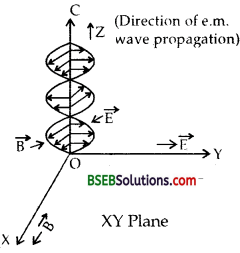Here the electromagnetic wave is travelling along Z-axis. Electric and magnetic field vectors $$\overrightarrow{\mathrm{E}}$$ and $$\overrightarrow{\mathrm{B}}$$ are normal to each other travelling along Y and X axis respectively (as per figure). Thus we conclude that with $$\overrightarrow{\mathrm{E}}$$ and $$\overrightarrow{\mathrm{B}}$$ are in XY plane and are perpendicular to each other.

Here v = frequency of e.m. wave 30 MHz = 30 x 106 Hz
C = Velocity of e.m. wave = 3 x 108 ms-1.
λ = ?
Weknow that
C = vλ .
∴ λ = $$\frac{C}{v}$$ = $$\frac{3 \times 10^{8}}{30 \times 10^{6}}$$ = 10m

Question 5.
A radio can tune in to any station in the 7.5 MHz to 12 MHz band. What is the corresponding wavelength band?
Here, frequency band of stations, v = 7.5 MHz to 12 MHz.
= 7.5 x 106 Hz to 12 x 106 Hz.
C = Velocity of radio waves = 3 x 108 ms-1.
λ = Wavelength band of stations = ?
We know that C = vλ .
∴ λ = $$\frac{C}{λ}$$

(i) When v = 7.5 MHz = 7.5 x 106 Hz.
Then
$$\frac{3 \times 10^{8}}{7.5 \times 10^{6}}$$ = 40 m

(ii) When v = 12 MHz = 12 x 106 Hz.
Then
$$\frac{3 \times 10^{8}}{7.5 \times 10^{6}}$$ = 25 m’
∴ Wavelength band of stations is 25 m to 40 m.Question 6.
A charged particle oscillates about its mean equilibrium position with a frequency of 109 Hz. What is the frequency of the electromagnetic waves produced by the oscillator?
We know that an accelerated particle radiates electromagnetic waves. An oscillating charge (example accelerated charge) oscillating with certain frequency produces oscillating electric field which produces oscillating magnetic field. These two oscillating fields regenerate each other. The frequency of electro-magnetic waves is equal to the frequency of oscillation of charge particle which is 109Hz here.

Question 7.
The amplitude of the magnetic field part of a harmonic electromagnetic wave in vacuum is B0 = 510 nT. What is the amplitude of the electric field part of the wave?
Here B0 = amplitude of magnetic field part of e.m. wave in vaccum = 510.nT = 510 x 10-9 T.
C = Velocity of e.m. waves in vacuum = 3 x 108 ms-1.
E0 = amplitude of electric field part of e.m. wave in vacuum = ?
We know that for electromagnetic waves,Question 8.
Suppose that the electric field amplitude of an electromagnetic wave is E0 = 120 N/C and that its frequency is v = 50.0 MHz. (a) Determine, B0 ω, k, and λ (b) Find expression for E and B.
Here, E0 = amplitude of electric field = 120 NC-1
v = frequency of e.m. wave = 50 MHz = 50 x 106 Hz.
C = velocity of em. wave = 3 x 108 ms-1.
(a) (i) B0 = ?,
(ii) k = ?
We know that(ii) We know that E0 K = B0 ω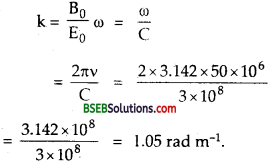(iii) λ is given by
λ = $$\frac{C}{υ}$$
= $$\frac{3 \times 10^{8}}{50 \times 10^{6}}$$ = 6m
(iv) ω = 27iv = 2 x 3.14 x 50 x 106 = 3.14 x 108 rad s-1.
Expressions for $$\overrightarrow{\mathrm{E}}$$ and $$\overrightarrow{\mathrm{B}}$$ are along Y and Z axis respectively.
.’. Equation for $$\overrightarrow{\mathrm{E}}$$ is.
$$\overrightarrow{\mathrm{E}_{\mathrm{y}}}$$ = E0 sin (kx – ωt) $$\hat{\mathbf{j}}$$
= 120 sin (1.05 x – 3.14 x 108 t) $$\hat{\mathbf{j}}$$ (NC-1)

Equation of B is :
$$\overrightarrow{\mathrm{B}_{\mathrm{z}}}$$ = B0 sin (kx – ωt) $$\hat{\mathbf{k}}$$ (T)
= 4 x 10-7 sin (1.05 x – 3.14 x 108 1) k (T)Aliter:
Equation for $$\overrightarrow{\mathrm{E}}$$ may be written as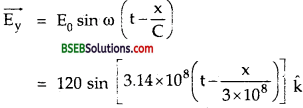Similarly equation for $$\overrightarrow{\mathrm{B}}$$ is given by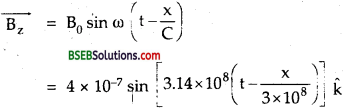Question 9.
The terminology of different parts of the electromagnetic spectrum is given in the text. Use the formula E = hv (for energy of a quantum of radiation: photon) and obtain the photon energy in units of eV for different parts of the electromagnetic spectrum. In what way are the different scales of photon energies that you obtain related to the sources of electromagnetic radiation?
Energy of photon is given by the relation, ‘
E = hv
E = $$\frac{\mathrm{hC}}{\lambda}$$ In SI
h = 6.62 x 10-34 Js = Plank’s constant.
C = 3 x 108 ms-1
= Velocity of e.m. wave in free space.

If λ, in metre, E in J. To convert E in electron volt we divide it by 1.6 x 10-19Different parts of e.m. Spectrum are :
1. y – rays : λ ranging from 10-10 m to less than 10-14 m.If λ = 10-14 m, Energy = 108 eV
Energy of y rays ranges between 104 to 108 eV.

2. x – rays: X from 10-8 m down to 10-13 m
For λ = 10-8 m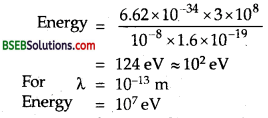Energy of X-rays ranges from 102 eV to 107 eV.

λ ranges from 4 x 10-7 m down to 6 x 1010 m.
For λ = 4 x 10-7 mEnergy of γ-rays lies 10° to 103 eV.

4. Visible Radiation : γ from 4000 Å to 7000 Å
For λ = 4000 Å = 4 x 10-7m.
As proved in ultra violet Radiation Energy = 3.1 eV = 100 eVλ ranging from 7 x 10-7 m to 7 x 10-4 m.
As in visible radiations for λ = 7 x 10-7 m energy is of the order of
100 eV and λ = 7 x 10-4m engry is $$\frac{1}{1000}$$ times that is of the order of 10-3eV
Energy of the order of 108 to 10-10 eV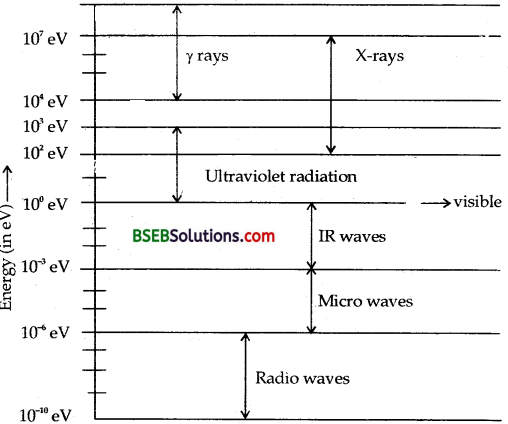6. Micro waves –
A has range From 1 mm to 0.3 m
For λ = 1 mm or 10-3 m energy is equal toFor A = 0.3 menergy = 4.1 x 10-6eV
Energy is of the order of 10-6 eV.

7. Radio waves : A, has range 1 m to few km.≈ 10-6 eV and for λ of the order of few km.
Energy of a photon that a source produces indicates the spacing of relevant energy levels of that source. e.g. X = 10-12 m corresponds to photo energy = 1.24 x 106 eV = 1.24 MeV. This indicates that nuclear energy levels (transition between which causes y-ray emission) are typically spaced by 1 MeV or so. Similarly a visible wavelength X = 5 x 10-7 m corresponds to photo energy = 2.5 eV. It means that energy levels i.e., transitions between which give visible radiation are typically spaced by a few eV.Question 10.
In a plane electromagnetic wave the electric field oscillates sinusoidally at a frequency of 2.0 x 1010 Hz and amplitude 48 V m-1 J
(a) What is the wavelength of a wave?
(b) What is the amplitude of the oscillating magnetic field?
(c) Show that the average energy density of the E field equals the average energy density of the B field. [c = 3x 108 ms-1].
Here, v = frequency of $$\overrightarrow{\mathrm{E}}$$ = 2.0 x 1010 Hz ,
E0 = amplitude of electric field = 48 Vm-1
C = 3 x 108 ms-1.
(a) λ = wavelength of the wave = ?
We know that, λ $$\frac{C}{v}$$ = $$\frac{3 \times 10^{8}}{2 \times 10^{10}}$$ =1.5 x 10-2 m.

(b) B0 = amplitude of oscillating magnetic field = ?
We know that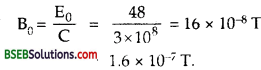(c) Let uE and uB be the energy density of $$\overrightarrow{\mathrm{E}}$$ field and $$\overrightarrow{\mathrm{B}}$$ field respectively.
To prove uE = uB.
We know that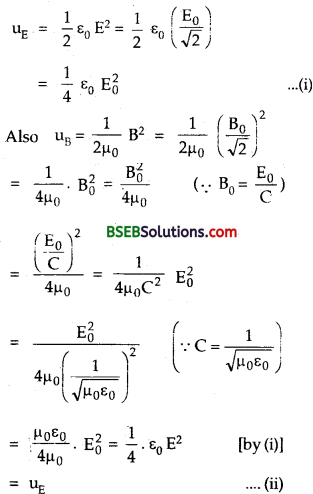∴From (i) and (ii), we get thai μE = μBHence proved:

Question 11.
Suppose that the electric field part of an electromagnetic wave in vacuum is E = {(3.1 N/C) cos [(1.8 rad/m)y + (5.4 x 108rad/s)} $$\hat{\mathbf{i}}$$
(a) What is the direction of propagation?
(b) What is the wavelength X?
(c) What is the frequency v ?
(d) What is the amplitude of the magnetic field part of the wave?
(e) Write an expression for the magnetic field part of the wave.
Here, $$\overrightarrow{\mathrm{E}}$$ = (3.1 NC-1) cos [(1.8 rad m-1) y + (5.4 x 108 rad s-1) t] $$\hat{\mathbf{i}}$$ ……..(1)
The given equation is of the type :
$$\overrightarrow{\mathrm{E}}$$ = E0 cos (ky + ωt) $$\hat{\mathbf{i}}$$ ……(2)
Comparing (1) and (2), we get
E0 = 3.1 NC-1
ω = 5.4 x 108 rad s-1.

(a) The electric field propagates along the direction of $$\hat{\mathbf{i}}$$ i.e., along
x – axis and the e.m. wave propagates along Y axis i.e. along $$\hat{\mathbf{i}}$$.

(b) λ = wavelength = ?
We know that co = 2πv = 2π$$\frac{C}{λ}$$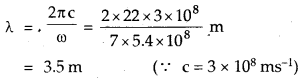(c) v = frequency =?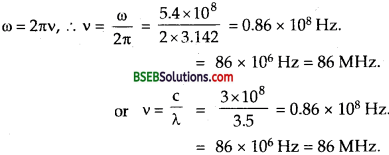(d) B0 = amplitude of magnetic field part of the wave =?
We know that C = $$\frac{\mathrm{E}_{0}}{\mathrm{~B}_{0}}$$(e) Expression for B = ?
It is given by
B = B0 cos (ky + ωt)
= (1.03 x 10-8T)

Direction of B :
We know that
$$\overrightarrow{\mathrm{C}}$$ = $$\overrightarrow{\mathrm{E}}$$ x $$\overrightarrow{\mathrm{B}}$$
i.e.,$$\overrightarrow{\mathrm{C}}$$ acts perpendicular to the plane of $$\overrightarrow{\mathrm{E}}$$ and $$\overrightarrow{\mathrm{B}}$$ Now $$\overrightarrow{\mathrm{C}}$$ acts along Y axis i.e., $$\hat{\mathbf{j}}$$, then $$\overrightarrow{\mathrm{B}}$$ will act along Z axis i.e., along $$\hat{\mathbf{j}}$$
$$\overrightarrow{\mathrm{B}}$$ = (1.03 x 10-8 T) cos [(1.8 rad m-1) + (5.4 x 108 rad s-1) t] k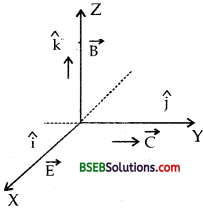Question 12.
About 5% of the power of a 100 W light bulb is converted to visible radiation. What is the average intensity of visible radiatidn.
(a) at a distance of 1 m from the bulb?
(b) at a distance of 10 m?
Assume that the radiation is emitted isotropically and neglect reflection.
Here, P = power of bulb = 100 W.
P’ = Power converted into visible radiation = 5% of P
= $$\frac{5}{100}$$ x 100 = 5 Js-1

(a) r = 1m, I = average intensity of visible radiation = ?
The bulb as a point source, radiates light in all directions uniformly. At a distance of 3 m, the surface area of the surrounding sphere is:
A = 4nr2
We know that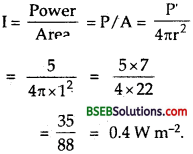(b) Here r = 10m, I = ?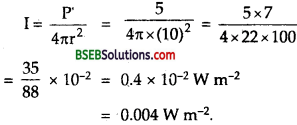Question 13.
Use the formula λmT = 0.29 cm K to obtain the characteristic temperature ranges for different parts of the electromagnetic spectrum. What do the numbers that you obtain tell you?
We know that every body at a given temperature T emits radiations of all wavelengths in certain region, thus we can say that it produces a continuous spectrum of wavelengths. For a black body, the wave length corresponding to maximum intensity of radiation at a given temperature T is given by the relation according to Wein’s displacement law as :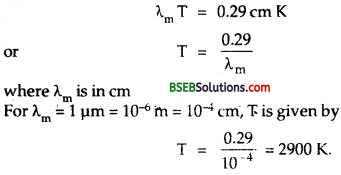Temperatures for other wavelengths can be found similarly. These numbers tell us the tempera hire rangps required for obtaining radiations in different parts of the e.m. spectrum. Thus to obtain visible radiations say having λm = 5000 A = 5000 x 10~10 m = 5 x 10~5 cm, the source should have a temperature, T given byIt should be noted that a body at lower temperature will also produce this wavelength but not with maximum intensity.

Question 14.
Given below are some famous numbers associated with electromagnetic radiations in different contexts in physics. State the part of the electromagnetic spectrum to which each belongs. .
(a) 21 cm (wavelength emitted by atomic hydrogen in interstellar space).
(b) 1057 MHz (frequency of radiation arising from two close
energy levels in hydrogen; known as Lamb shift.)
(c) 2.7 K [temperature associated with the isotropic radiation filling all space-thought to be a relic of the ‘big-bang’ origin of the universe.
(d) 5890 A – 5896 A [double lines of sodium]
(e) 14.4 keV [energy of a particular transition in 57Fe nucleus associated with a famous high resolution spectroscopic method (Mossbauer spectroscopy)].
(a) Here, λ = 21 cm. This wavelength of the electromagnetic waves corresponds to radio waves (which have short wavelength or high frequency end).
(b) Here v = 1057 MHz; so the corresponding wavelength is given by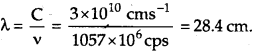Thus this wavelength also corresponds to short wavelength end i. e., radiowaves.
(c) Here T = 2.7 K
∴ According to wein’s displacement law,This wavelength corresponds to microwave region of the electromagnetic spectrum.
(d) λ – 5890 Å – 5896 Å which is doublet of sodium light and it lies in the visible region (yellow) of the electromagnetic spectrum.
(e) Here, E = energy = 14.4 KeV = 14.4 x 103 x 1.6 x 10-19 J (∴ 1eV = 1.6x 10-19J)
Also we know thatwhich corresponds to X-ray region (i.e., soft y-ray region) of the electromagnetic spectrum.

Question 15.
(b) It is necessary to use satellites for long distance TV
transmission. Why?
(c) Optical and radiotelescopes are built on the ground but X- ray astronomy is possible only from satellites orbiting the earth. Why?
(d) The small ozone layer on top of the stratosphere is crucial for human survival. Why?
(e) If the earth did not have an atmosphere, would it average surface temperature be higher or lower than what it is now?
(f) Some scientists have predicted that a global nuclear war on the earth would be followed by a serve ‘nuclear winter’ with a devastating effect on life on earth. What might be the basis of this prediction?
(a) Long distance radio broadcasts use short-wave bands because ionosphere reflects the waves lying in the frequency range of short wave band i.e., the waves in these bands.

(b) Yes, it is necessary to use satellites for long distance T.V. transmission because T V- signals being of high frequency are not reflected by the ionosphere. Therefore”to reflect the signals back to earth’s surface for long distance transmission, satellites are used.(c) Optical and radio waves can penetrate the atmosphere where— as X-rays being of much smaller wavelength are absorbed by the earth’s atmosphere which is transparent to visible light and radiowaves and opaque to X-rays. That is why we can work with optical and radio telescope on earth’s surface but X-ray astronomy is possible only from the sakllìtes orbiting above the earth’s atmosphere.

(d) The small ozone layer present on the top of the stratosphereabsorbs the ultraviolet radiations, X-rays, y-rays from the sun along with the cosmic rays which are dangerous and cause genetic damage to the Livthgells and tissues preventing them from reaching the earth’s surface and thus helps in the survival of the life.

(e) Due to green house effect, the temperature of earth’s surface is raised in the presence of the atmosphere. In the absence of the atmosphere, the heat received by the earth during day time will be completely lost during night. Hence the temperature of earth would be lowered because of the absence of green House effect.

(f) The clouds produced by a global nuclear war would perhaps cover most parts of the sky preventing the solar light from reaching many parts of the globe and thus it may cause a severe nuclear winter.

### Bihar Board Class 12 Physics Chapter 8 Electromagnetic Waves Additional Important Questions and Answers

Question 1.
Define displacement current.
It is defined as the current produced due to the changing electric field or electric flux between the plates of the capacitor.

Question 2.
Which part of the electromagnetic spectrum is used in operating a RADAR? What is its wavelength range?
A radar is. operated in the microwave region of the electromagnetic spectrum. Its wavelength range is 1O m to 0.3 m.

Question 3.
Give one use of ¡nfra-red rays.
mfra-red ray photographs are used for weather forecasting.

Question 4.
Define e1ectzomagnetic spectrum. .
It is defined as an orderly arrangement of radiations in the form of distinct groups having widely different properties according to
the wavelength or frequency.

Question 5.
The charging current for a capacitor is 0.25 A. What ¡s the displacement current across its plates?
We know that the displacement current is always equal to the conduction current. As conduction current is equal to 0.25 A, so the displacement current is also 0.25 A.

Question 6.
Name the sources of magnetic field.
Conduction current and displacement currents are the sources of magnetic field.

Question 7.
What do electromagnetic waves consist of?
The e.m. waves consist of oscillating electric and magnetic fields,Question 8.
Why is the transmission of signals using ground waves restricted to frequency less than about 1500 KHz?
The transmission of signals using ground waves is restricted to frequency less than about 1500 KHz because the absorption of signals by earth’s surface increases above this frequency.

Question 9.
Why microwaves are better carriers of signals for long range transmission than radio waves?
Microwaves are better carriers of signals for long range transmission than radio waves because micro waves have much shorter wavelength than radio waves thus they suffer minimum deviation due to obstructions and can be beamed direct to the target.

Question 10.
Give the ratio of the velocities of light rays of wavelengths 4000 A and 8000 A in vacuum. Why?
The ratio is one because the velocity of light is independent of the wavelength in vacuum.

Question 11.
Write the following radiations in an descending order in respect of their frequencies: X-rays, micro-waves, ultra violet rays and radio waves.
The required descending order is as follows:
X-rays, ultra violet rays, microwaves and radio waves.

Question 12.
Wavelength of the electromagnetic radiation is doubled. What will happen to the energy of photons? ,
We know that for em radiations, C = vλ
v = $$\frac{C}{λ}$$
If λ is doubled, then v becomes half as C remains constant. Also we know that E = hv i.e., E ∝ v, thus the energy E becomes half.

Question 13.
Why microwaves are used in operating a ‘radar’?
The microwaves are used in operating radar because they can travel in a particular direction in the form of a beam due to their very small wavelength.

Question 14.
Which part of the electromagnetic spectrum has largest penetrating power?
y-rays has largest penetrating power.Question 15.
Arrange the following electromagnetic radiations in the descending order of their wavelengths: y-rays, infra red rays, red light, yellow light, radib waves.
The required descending order is as follows : radiowaves, infrared rays, red light, yellow light, y-rays.

Question 16.
Which em radiations are used for viewing objects through haze and fog? ‘
Infra red rays or radiations are used for viewing objects through haze and fog.

Question 17.
Arrange the following radiations in an ascending order in , respect of their frequencies: x-rays, microwaves, y-rays, UV rays, radio waves.
The ascending order in terms of their frequencies is radio waves, micro waves, UV rays, X-rays, y-rays.

Question 18.
Give one medical use of UV rays.
UV rays are used for sterilising surgical instruments.

Question 19.
Write down the relation between the amplitude of electric and magnetic fields in free space for an electromagnetic wave.
The amplitudes of electric field (E0) and field (B0) are related to each other in free space for an electromagnetic wave as given below:
C = $$\frac{\mathrm{E}_{0}}{\mathrm{~B}_{0}}$$
where C = speed of light in vacuum.

Question 20.
Both radio waves and y-rays are e.m. waves in character, transverse in nature and have same speed in vacuum. In what respects then they differ from each other?
(i) The radiowaves have atomic origin while the y-rays have nuclear origin.
(ii) The y-rays have large penetrating power while the radiowaves have small penetrating power.

Question 21.
Which of the following rays belong to e.m. spectrum?
a-rays, P-rays, y-rays, cathode rays, X-rays, UV rays, micro waves, ultrasonic waves, radiowaves, infrared rays.
The rays which belong to the e.m. spectrum are:
y-rays, x-rays, UV-rays, microwaves, radiowaves, infrared rays.

Question 22.
Arrange the e.m. waves given in Q. 23 above in the ascending order of their frequency?
Radio waves, micro waves, infra red rays, UV rays, X-rays and y-rays. ’

Question 23.
Which law asserts that the electric field lines cannot form a closed path?
Gauss’ law asserts that the electric field lines cannot form a closed path.

Question 24.
What is common between different types of e.m. radiations?
(i) They all are of transverse nature;
(ii) They travel with the same speed in vacuum i.e., with C = 3 x 108 ms-1.

Question 25.
Give the frequency range of T.V; signals.
The T.V. signals are of high frequencies having frequency range 100 MHz to 200 MHz.Question 26.
From which layer of atmosphere radio and microwaves are reflected back?
The radio and micro waves are reflected back from the ionosphere layer of the atmosphere.

Question 27.
For which frequency of light, the eye is most sensitive?
The eye is most sensitive to the yellow light of wavelength X = 5890 x 10-8 m which has frequency given by :Question 28.
Calculate the time period of the radiation in Q. 27.
From Q. 27, v = 5.09 x 1014 Hz.
If Tbe the time period of the light to which our eye is most sensitive,
thenQuestion 29.
Name the main parts of e.m. spectrum.
The main parts of e.m. spectrum arey-rays, X-rays, L’V-rays, Visible light, IR rays, heat radiations, microwaves and radiowaves.

Question 30.
Write the frequency limit of visible range of em. spectrum in kHz.
We know that the wavelength range of visible part of e.m. spectrum is 4000 Å to 8000 Å.
∴ The corresponding frequency range of visible spectrum is 4 x 1014 Hz to 7.7 (* 8) x 1014 Hz. .

Question 31.
How does the frequency of a beam of UV light changes when it goes from air into glass?
There is no effect on the frequency of UV light.

Question 32.
Write the expression for speed of electromagnetic waves in free space?
The speed of e.m. waves in free space is given by :
C = $$\frac{1}{\sqrt{\mu_{0} \varepsilon_{0}}}$$Question 33.
Define earth’s atmosphere.
It is defined as the gaseous envelope surrounding the earth.

Question 3.
State some applications of radio waves and microwaves.

• Radiowaves are used for wireless communication pi irposes.
• Microwaves are used in the study of atomic and molecular structure.
• Microwaves are used in radar systems and in long distance telephone communication systems.
• Microwave ovens which are used for cooking also work by micro waves.

Question 4.
State some properties of infra-red rays.

• They are e:m. waves and travel with the speed of light in vacuum.
• They obey laws of reflection and refraction.
• They affect a photographic plate.
• They can be polarised.
• They can produce interference.
• They are scattered less as compared to visible radiations by the atmosphere under the conditions of fog and smoke.
• Their energy is convered into molecular vibrations when they are absorbed by the molecules.

Question 5.
State some applications of y-rays.

• They are used in radiotherapy to treat tumours and cancer.
• They (soft y-rays) are used to kill micro organisms in food industry so as to preserve food stuffs for a longer time.
• They are used to produce nuclear reactions.

Question 6.
Mention some applications of IR-rays.

• They are used to treat muscular strains.
• They are used to provide electrical energy to satellites by – using solar cells.
• They are used to produce dehydrated fruits.
• They are used in solar water heaters and cookers.
• They are used to take photographs during the conditions of fog and smoke.
• IR photographs are used for weather forecasting.
•  Infrad-red (IR) raysfrom the sun keep the earth warm and hence help to sustain life on earth. *

Question 7.
Mention some uses of UV-rays.

• They are used to check the mineral samples by making use of its property of causing flourescence. .
• They are used in burglar alarms as they can cause photoelectric effect.
• They are used to sterilize surgical instruments as they can destroy bacteria.
• UV absorption spectra is used in the study of molecular structure.Question 8.
State some properties of UV rays.

• They obey the laws of reflection and refraction.
• They can undergo interference and can be polarised.
• They can cause flourescence in certain materials.
• They can affect photographic plates.
• They can synthesize vitamin D when the skin is exposed to the sunlight.
• They cause emission of photoelectrons when are allowed to fall on metals.
• They cannot pass through glass/
•  Rock salt, fluorite and quartz ark transparent to UV light.

Question 9.
Mention some properties of X-rays.

• They can undergo reflection, refraction, interference diffraction and polarisation.
• They affect photo graphic plates.
• They cast shadows of the objects falling in their path due to the reason that they travel in a straight line.
• They can penetrate the materials that are opaque to visible or UV light.
• They can cause photoelectric effect.
• They can cause flourescence in certain materials like zinc sulphide, calcium tungstate etc.
• They ionise the gas through which they pass.
• They produce secondary X-rays when fall on certain metals. The secondary X-rays are accompanied by fast moving electrons.
• They are em. waves of very short wavelength and thus earth’s atmosphere is opaque for X-rays.

Question 10.
Prove that ID = $$\varepsilon_{0} \frac{\mathbf{d}}{\mathrm{dt}}$$ (øE) Where the symbols have their usual meanings.
Proof: We know that ID = I ………..(1)
Also we know that I = $$\frac{dq}{dt}$$ ……….(2)
and according to Gauss’s law,
E = $$\frac{\mathrm{q}}{\varepsilon_{0} \mathrm{~A}}$$
or q = ε0 AE ………(3)
∴ From (1), (2) and (3), we getHence proved.

Question 11.
What conclusions can be drawn about the displacement current and conduction current?
(i) Both the displacement current and conduction current are discontinuous individually but the two currents together has the property of continuity along any closed path.
(ii) The displacement current like the conduction current is also a source of magnetic field.
(iii) The displacement current is always produced due to the change in electric flux or electric field with time between the plates of the capacitor.
(iv) Tire two currents are always equal to each other.
(v) ID exists between the plates of a capacitor while Ic (conduction current) in the wire connecting the plates with the source of e.m.f.Question 12.
Mention some applications of X-rays.

• They are used in surgery for detectibn of fractures, diseased organs etc.
• They are used in radio therapy.
• They are used to detect explosives, opium, silver in the body of smugglers by detective departments.
• They arc used to distinguish real diamonds, gems etc. from artificial ones.
• They are used in industry for detecting the pearls in oysters and for testing the uniformity of insulating materials anc’ homogeneity of timber.
• They are also used in scientific research to investigate the structure of crystaLs. arrangement atoms etc.

Question 13.
Define and explain green House effect.
It is defined as the process of heating of earth’s atmosphere dueto infrared rays emitted by objects on earth and reflected back by CO2 layers in the atmosphere. Sun’s radiations on reaching earth heat earth and 1 R radiations are radiated by earth which are reflected back by the gases in the lower layers of atmosphere and the clouds. Thus radiations from the earth is unable to cross the lower atmosphere which reflects it back. This helps in keeping he earth’s surface warm at night and is called green House effect.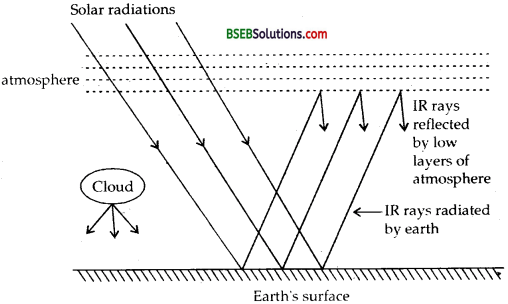Question 14.
Write down four Maxwell equations.
Four basic laws of electricity and magnetism i.e., Guass’s law in electrostatic, Guass’s law in magnetism, Faraday’s law of electromagnetic induction and Maxwell-Ampere’s circuital law are called Maxwell’s equations. These are(iii) e = – $$\frac{\mathrm{d} \phi_{\mathrm{B}}}{\mathrm{dt}}$$ where e is induced erftf and is line integral of electric field and can be written as $$\oint \vec{E} \cdot \overrightarrow{d l}$$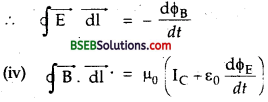Question 15.
Define conduction current. Why did Maxwell introduce the concept of displacement current?
Conduction Current – It is defined as the current flowing in the circuit through the connecting wires. Consider a capacitor C being charged by current IC through a battery. It is clear from the figure that during the charging of the capacitor, current IC flows from right plate of the capacitor to its left plate and no conduction current (I) flows in between the plates of the capacitor.To avoid this discrepency, Maxwell introduced a new concept of displacement current (ID) and attributed its origin to the varying electric field between the plates Of the capacitor. It was done to show that current flowing outside the capacitor (i.e., Ic; is always equal to the current flowing inside the capacitor (i.e., IC). According to Maxwell, conduction current I flows into the left plate of the capacitor and an equal displacement current (ID) flows out ‘from it to the right plate of the capacitor.Question 16.
Explain flow Ampere’s circuital law was modified by Maxwell?
According to Maxwell, the contradiction arising in Ampere’s circuital law is due to the assumption of discontinuity of current between the plates of the capacitor. He proposed the concept of displacement current (ID) where there is no conduction current (IC) ID flows. As the capacitor charges, the electric field between the plates of the capacitor increases and gives rise to flow of displacement current in between the plates of the capacitor.
Thus Ampere’s circuital law ($$\oint \overrightarrow{\mathrm{B}} \cdot \overrightarrow{\mathrm{dl}}=\mu_{0} \mathrm{I}_{\mathrm{C}}$$) was modified to:
$$\oint \overrightarrow{\mathrm{B}} \cdot \overrightarrow{\mathrm{d} 1}=\mu_{0}\left(\mathrm{~L}_{\mathrm{C}}+\mathrm{I}_{\mathrm{D}}\right)$$

Question 17.
Draw a labelled diagram of Hertz experiment for generation of e.m. waves. How they were detected?
The diagram is shown in the figure given here. It consists of two metal plates P1 and P2 connected to a source ofhigh d.c. voltage (i.e„ induction coil). The plates P1 and P2 are connected to small spheres S1 and S2 having a narrow gap.The induction coil produce^ large potential difference across the plates after every time interval of nearly 1000 µs. Due to high potential difference between the plates, theVair in the gap gets ionized and continuous spark is produced between the gap.
The frequency of oscillation of the charge is given by
v = $$\frac{1}{2 \pi \sqrt{L C}}$$
Where C = Capacitance provided by metal plates.
L = inductance of the circuit.
The frequency of oscillation of electric charge was found to be 5 x 107 Hz. After every 103 ps, electric discharge between the spheres S; and S2 is produced and each time a new pulse of e.m. wave moves with the speed of light in space and is detected by the detector D which is in the form of unclosed metallic ring having small spheres C and C’ with some gap. It is held in such a position that the magnetic field produced by oscillating charge or current is normal to the plane of ring. The oscillating magnetic field linked with the ring produces large induced e.m.f. between C and C’ which causes a spark to appear at spheres C’ and C. Wavelength of the electromagnetic waves produced is given by
λ = $$\frac{C}{v}=\frac{3 \times 10^{8}}{5 \times 10^{7}}$$ = 6mQuestion 18.
Why radio waves are diffracted around buldings while light waves are not diffracted.
For diffraction to take place, the wave length of light or radiowaves should be comparable to the size of the obstacle or aperture. Short radiowaves have wavelength of the order of the size of the buildings, so they are easily diffracted while light having very short wave length cannot be diffracted.

Question 19.
Why is it that induced electric field due to changing magnetic flux is easily observable than the induced magnetic field due to changing electric field?
Induced electric field due to changing magnetic flux is sufficient and can be increased by taking coil of large number of turns so it is easily observable. But induced magnetic field is produced due to displacement currents and it is very small in magnitude. Induced magnetic field can be increased by increasing displacement currents by charjging angular frequency of current in an a.c. circuit.

Question 1.
Using Ampere’s modified law, calculate the magnetic field between the plates of a capacitor at a point.
Let R be the radius of circular plates of the capacitor.
IC = Conduction current flowing in the connecting wires.
ID = displacement current between the plates.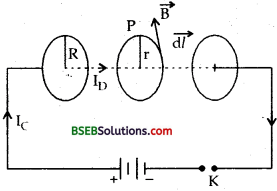Let r be the distance of the point P between the plates at which the magnetic field is to be calculated. Draw a circle of radius r between the plates. If $$\overrightarrow{\mathrm{B}}$$ be the magnetic field at P then it will act tangentially to the circle.
∴ According to Ampere’s modified law,
$$\oint \overrightarrow{\mathrm{B}} \cdot \overrightarrow{\mathrm{dl}}=\mu_{0}\left(\mathrm{I}_{C}+\mathrm{I}_{\mathrm{D}}\right)$$
Now IC = 0 between the plates of the capacitor.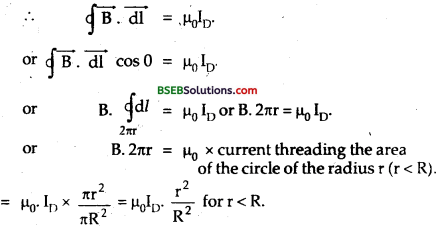Numerical Problems

Question 1.
In a plane em. wave the electric field oscillates with a frequency of 2 x 10 s-1 and an amplitude of 40 Vm-1. Calculate: (a) The – wavelength of the wave, (b) energy density associated with the electric field.
Here, v = 2 x 1010 s-1
= frequency of oscillation of $$\overrightarrow{\mathrm{E}}$$
C = vol. of light =3 x 108 ms-1
E0 = amplitude of electric field
= 40 Vm-1
Er.m.s = $$\frac{E_{0}}{\sqrt{2}}=\frac{\sqrt{2}}{2} \mathrm{E}_{0}$$ = $$\frac{E_{0}}{\sqrt{2}}=\frac{\sqrt{2}}{2} \mathrm{E}_{0}$$ = $$\sqrt{2} \times \frac{40}{2}=20 \sqrt{2}$$

Wavelength of the wave = ?
We know that C = v λ
λ = $$\frac{C}{v}=\frac{3 \times 10^{8}}{2 \times 10^{10}}$$ = 150 m
= 1.5 x 102m.(b) UE = ener tdensity due to electric field =?
We knöw that ‘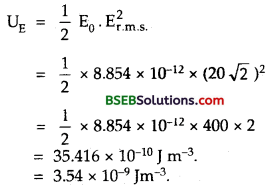Question 2.
A capacitor is made of two circular plates each of radius 12 cm and separated by 5 x 10-3 m and it is charged by a steady current of 0.15 A. Use Ampere’s modified law to calculate the magnetic field between the plates at a point:
(a) on the axis.
(b) 6.5 cm from ,fhe axis.
(c) 15 cm from the axis.
Calculate theinaximum value of the magnetic field.
Here, r = 12 cm = 12 x 10-2 m.
A = Area of each plate = πr2 = π x (12 x 10-2)2 = π x 144 x 10-4m2
d = 5 x 10-3 m.
I = 0.15 A.
We know that magnetic field at a point a distance x from the axis of a circular plate capacitor is given by
B = $$\frac{\mu_{0}}{2 \pi} \cdot \frac{I_{D} x}{r^{2}}$$ = $$\frac{\mu_{0} I_{D}}{2 \pi x} \times \frac{x^{2}}{r^{2}}$$ …………(1)
If x>>r,then B = μDID/2πx ……….(2)
(a) Here, x = 0
∴ from equation (1), B = 0

(b) Here, x 6.5 cm 6.5 x 10-2 m.
From (1),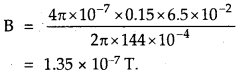(c) Here, x = 15 cm = 15 x 10-2 m.
∴ From (2),

(d) When x ≥ r, then the magnetic field is given by(d) When x ≥ r, then the magnetic field is given byB will be maximum if, r = 12 cm = 12 x 10-2 m.Question 3.
The e.m. wave travel in a medium with a speed of 2 x 108 ms-1. The relative permeability of the medium is 1. Find the relative permitivity.
Here, v = speed of e.m. wave in the medium = 2 x 108 ms-1.
µr = relative permeability of the medium = 1
εr = relative permitivity of the medium = ?
C = speed of light in vacuum = $$\frac{1}{\sqrt{\mu_{0} \varepsilon_{0}}}$$ = 3 x 108 ms-1.
We know that the speed of light in a medium is given by
u = $$\frac{1}{\sqrt{\mu \varepsilon}}$$ = ……………..(1)
Where |i and e are the absolute permeability and absolute permitivity of the medium and are given by
μ = μ0μr and e = ε0 εr ………..(2)
∴ From (1) and (2), we getQuestion 4.
Electromagnetic waves of frequency 5 x 1014 Hz are passed through a liquid. The wavelength of the waves in liquid is measured to be 4.5 x 10-7 m. Calculate : (a) the wavelength of the e.m. waves in vacuum (b) velocity of e.m. waves in the liquid, (c) refractive index of the liquid. Given: Velocity of e.m. waves in vacuum = 3 x 108 ms-1.
Here, v = frequency of e.m. waves = 5 x 1014 Hz.
λ liquid = wave length of waves in liquid = 4.5 x 10-7 m.
c = Velocity of e.m. waves in vacuum = 3 x 108 ms-1(a) λ vacuum = Wavelength of em. waves in vacuum
= ?
Using the relation,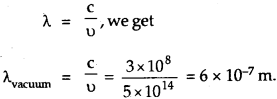(b) v = Velocity of e.m. waves in liquid = ?
Using the relation, υ = v λ liquid, we get
υ = 5 x 1014 x 4.5 x 10-7
= 2.25 x 108 ms-1

(c) μ = refractive index of the liquid = ?
We know thatQuestion 5.
Calculate the energy in watt-house of electromagnetic waves of wavelength 3000 Å. Given, h = 6.63 x 10-34JS. *
Here, λ = wavelength of e.m. waves = 3000 Å
= 3000 x 10-10 m.
h = 6.63 x 10-34 JS
E = ? (in wh).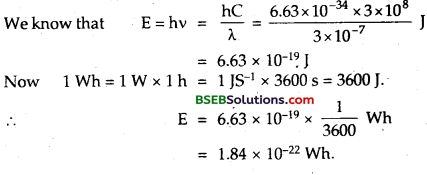Question 6.
What are the dimensions of $$\frac{1}{2}$$ ε0 E2 ?
For dimensions of s0 ε0, we use the formulaWriting dimensions of all quantities on RHS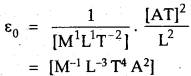For dimensions of E, we use formula F = qE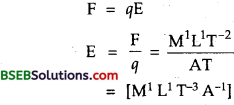∴ Dimensions of $$\frac{1}{2}$$ ε0E2are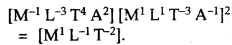Question 7.
Green light of Hg has a wave length 5.5 x 105cm. What is the
(a) frequency in MHz and period in ps in vacuum
(b) What is wave length in glass if refractve index of glass is 1.5 ? Given C = 3 x 108 ms-1.Question 8.
The rate of change of electric field in the region of the plates of capacitor is 1.5 x 1012V-1m4 s-1. If radius of circular plates of capacitor is 55 mm, what is displacement current?
ID = ? We know that ID = ε0 $$\frac{d \phi_{\mathrm{E}}}{d t}$$
But øE = EA
∴ ID = ε0 $$\frac{d}{dt}$$(EA) = ε0A $$\frac{dE}{dt}$$
Here r = 55mm =55 x 102m = 5.5 x 10-2m
and A = πr2 x $$\frac{22}{7}$$ (5.5 x 102)2
= 95.07 x 10-4m2
And $$\frac{d}{dt}$$ = 1.5 x 1012 Vm-1s-1
Also ε0 = 8.854 x 10-12 SI units
ID = 8.854 x 10-12 x 1.5 x 1012 x 95.07 x 10-4
= 126.149 x 10-3A = 126 mA.Question 9.
A parallel plate capacitor of. plate separation 2 mm is connected in an electric circuit having source voltage 400 V. What is the value of the displacement current for 10″6 s if the plate area is 60 cm2?
Here, d = spacing between the plates = 2 mm = 2 x 10-3 m
V = 400 V .
t = 10-6 s
A = 60 cm2 = 60 (10-2 m)-2 = 60 x 10-4 m2.
ID = ?

We know thatQuestion 10.
An observer is 1.8 m from an isotropic point light source whose power is 250 W. Calculate the r.m.s. values of the electric and magnetic fields due to the source at the position of the observer.
Here, P = power of light source = 250 W.
r = distance of the observer from the source = 1.8 m.
∴ Surface area of the surrounding sphere with the light source at centre is given by
A = 4πr2 = 4π x (1.8)2 m2.
If I be the intensity of the e.m. wave, then Er.m.s= ? Br.m.s = ?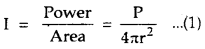Also we know that
I = (average enegry density of $$\overrightarrow{\mathrm{E}}$$)Question 11.
A beam of light travelling along x-axis is described by the electric field, Ey = 600 Vm-1 sin ω (t – $$\frac{x}{C}$$)
Calculate the maximum electric and magnetic force on a charge = 2e, moving along y-axis with a speed of 3.0 x 107 ms-1, where e = 1.6 10-19 C.
Here, Ey = 600 Vm-1 sin ω (t – $$\frac{x}{C}$$)
Ey = 600 Vm-1
q = 2e = 2 x 1.6 x 10-9 C
C = 3 x 108 ms-1 υ = 3.0 x 107 ms-1
∴ B0 = $$\frac{E_{0}}{C}=\frac{600}{3 \times 10^{8}}$$
= 2 x 106 T which acts along z-axis.
Thus maximum electric force is given by
Fe = qE0 = 2e E0 = 2 x 1.6 x 10-19 x 600
= 1.92 x 10-16 N.
The maximum magnetic force is given by
Fm = qυB0 = 2eυB0.
= 2 x 1.6 x 10-19 x 3 x 107 x 2 x 10-6N = 1.92 x 10-17N.Question 12.
Find the energy stored in a 90 cm length of a laser beam operating at 10 mW.
Here P = 10 mW = 10 x 10-3 W.
Let t = time taken by laser beam to move through a distance of 90 cm is given by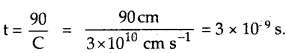The energy contained in 90 cm length of laser beam is given by:
U = P.t = 10 x 10-3 x 3 x 10-9 J
= 30 x 10-12 J.

Question 13.
The charging current across the plates of a capacitor is 0.5 A. Calculate the displacement current across its plates.
Here, I = 0.5 A, ID = ?
We know that
ID = ε0 $$\frac{\mathrm{d} \phi_{\mathrm{E}}}{\mathrm{dt}}$$
where øE = EA, A = area of plates.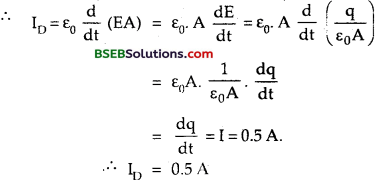Question 14.
How would you establish an instantaneous displacement current of 2A in space between the plates of 2 μF capacitor?
Here C = 2μF = 2 x 10-6 F,
ID = 2A
Also we know thatSo ID of 2A can be set up by a rate of charge of potential across the plates of capacitor by 10-6 Vs-1

Question 15.
A 100 pF capacitor is connected to 9230 V, 50 Hz a.c. source. What is the r.m.s. value of conduction current?
Here, C = 100pF = 100 x 10-12 F = 10-12F, Er.m.s =230V, υ = 50 Hz.
Ir.m.s = r.m.s. value of conduction current = ?
We know that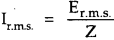When Z impedance of the circuit = Xc = $$\frac{1}{ωC}$$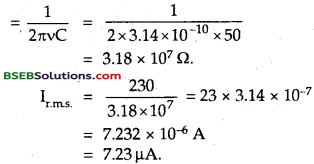Fill In The Blanks

Question 1.
The varying electric field between the plates of the capacitor gives rise to and hence is also produced.
Displacement current, magnetic field.

Question 2.
………… produces magnetic field in the same way as does the conduction current.
Displacement current.

Question 3
…………. are transverse in nature.
Electro
magnetic waves.

Question 4
………….. is responsible for the optical effects due to an electromagnetic radiation and hence it is also called as ………..
Electric vector, light vector.

Question 5.
The ratio W/C is also called as the magnitude of for an electromagnetic wave.
Propagation vector (k).Question 6
…………. of electromagnetic waves is their inherent characteristic and does not change when it travels from one medium into another but its changes.
Frequency, wavelength.

Question 7.
………….. produces heat when absorbed by the matter and are used in radio astronomy and radar system.

Question 8.
When allowed to fall on matter ………….. rays produce an increase in temperature.
infrared.

Question 9.
……….. are used for producing dehydrated fruits.
Infrared rays.

Question 10.
………… and …………. cause flourescence in certain substances.
Ultra-violet rays and X-rays.

Question 11.
………….. rays have higher penetrating power and small
Gamma, ionising power.

Question 12.
…………. helps to keep the earth’s atmosphere warm by preventing the escape of ………… from the earth’s surface and traps them.
Ozone layer, infrared rays.

Question 13.
The …………. is the main source of electromagnetic radiation.
Sun.Question 14.
………….. layer absorbs most of the UV radiation and other harmful radiations of lower wave lengths.
Ozone layer.

Question 15.
The ozone layer converts ………… radiation to infra-red.
Ultraviolet (UV).

Question 16.
………… rays are of nuclear origin while X-rays are of …………